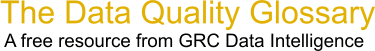Choose Index below for a list of all words and phrases defined in this glossary.

# Mean

index | Index

## Mean - definitions

Mean : The average of a set of values, usually calculated to one place of decimals more than the original data.

[Category=Data Quality ]

Source: Larry English, http://www.iaidq.com/main/glossary.shtml, 02-Feb-2009 10:31

These advertisers support this free service

Mean - The arithmetic mean is a measure of central tendancy that is calculated by dividing the sum of observed values by the number of observations. For example: the arithmetic mean for age would be 30 for the following set of observations: John = 20, Joyce = 30, Jim = 40

[Category=Database Management ]

Source: NextMark, 19 July 2009 10:48:27, https://www.nextmark.com/resources/glossary-of-terms/ ?glossaryTermId=a0800000000FVTJAA4Mean - A measure of central tendency; the arithmetic average of all measurements in a data set.

[Category=Quality ]

Source: American Society for Quality, 04 October 2010 07:39:33, http://www.asq.org/glossary/Mean - The mean is the average data point value within a data set. To calculate the mean, add all of the individual data points then divide that figure by the total number of data points.

[Category=Data Quality ]

Source: iSixSigma, 03 February 2011 09:05:52, http://www.isixsigma.com/index.php?option=com_glossaryMean (arithmetic) - The sum of all the values of a set of measurements divided by the number of values in the set; a measure of central tendency

[Category=Quality Assurance ]

Source: Massachusetts Department of Environmental Protection, 23 March 2011 09:09:06, http://www.mass.gov/dep/cleanup/laws/glossa01.htmmean - [mathematics] The average for a set of values, computed as the sum of all values divided by the number of values in the set.

[Category=Geospatial ]

Source: esri, 08 June 2012 09:04:00, http://support.esri.com/en/knowledgebase/GISDictionary/term/abbreviationData Quality Glossary.  A free resource from GRC Data Intelligence. For comments, questions or feedback: dqglossary@grcdi.nl